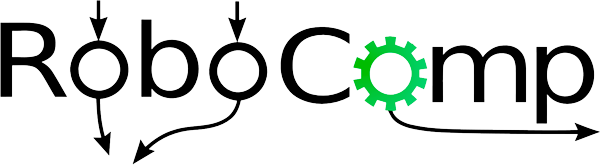# A usecase for the previously built toolkit

The basic framework for the tool kit was done in the intial phase after that some remaining future works were left that are done, namely

• Graceful exit: Each component is made to be serially killed. We used a signal handler, when the kill signal is received by each component the handler kills the component. The signal sent to each components is SIGTERM.
• Asking for the contributor’s name at startup of the GUI so the files can be saved with that name.

## The usecase:

One way we used the data collected from the toolkit is by training graph based machine learning models to predict the robot’s path trajectory to navigate in the social setting. We implemented and trained a series of Graph Neural Nets also different variations of graph convolutional network, and some combinations of different GCNs to find the net which can provide with the most accuracy for the robot’s path prediction. Some of the nets that were trained are:

• Chebyshev Spectral Graph Convolutional Network
• Gated Graph Convolutional Network
• Graph Convolutional network
• Relational Graph Convolutional network
• Self-Attention Graph Pooling Graph Convolutional network
• Topology Adaptive Graph Convolutional Networks
• Interwined RGCN and GAT
• Parallel RGCN and GAT

Rishabh